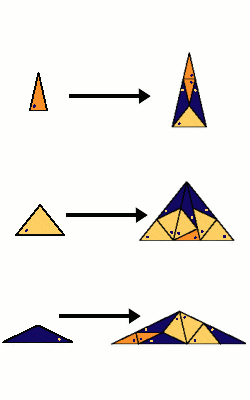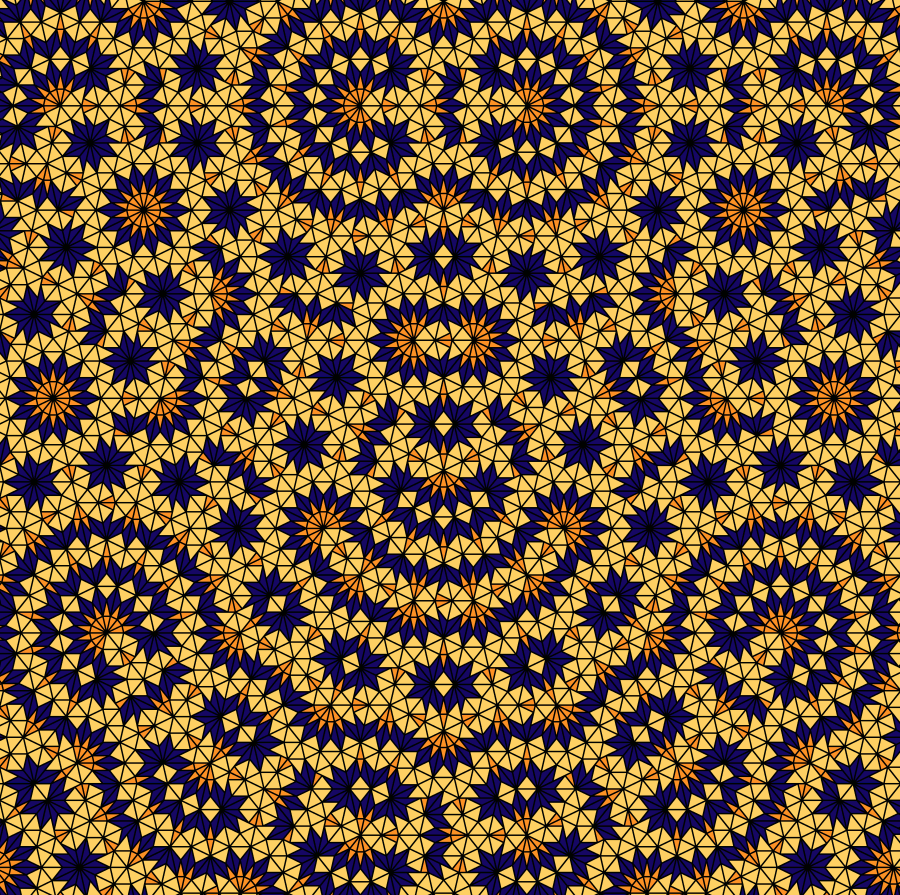## Danzer's 7-fold

### Info

A substitution tiling with three triangles as prototiles, based on 7-fold symmetry. The four different edge lengths occurring are $\sin(\frac{\pi}{7})$, $\sin(\frac{2\pi}{7})$, $\sin(\frac{3\pi}{7})$, $\sin(\frac{2\pi}{7}) + \sin(\frac{3\pi}{7})$, The inflation factor is $1+{\sin(\frac{2\pi}{7})}/{\sin(\frac{\pi}{7})}$ , which is not a PV number.

There are simple matching rules for the tiling. In fact, the list of all vertex stars occurring in the substitution tiling serves as one. This is stated in [ND96], but never really published, up to my knowledge. The mentioned paper focusses on different tilings.

### Substitution Rule### Patch### References

[ND96]
Nischke, K-P and Danzer, L
A construction of inflation rules based on $n$-fold symmetry
Discrete and Computational Geometry 1996, 15,2, pp. 221-236, 96j:52035